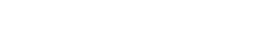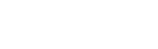Z-Score标准化：将原始数据映射到均值为0、标准差为1的分布上## 为什幺要标准化/归一化？## 哪些机器学习算法需要标准化和归一化

2）概率模型、树形结构模型不需要归一化，因为它们不关心变量的值，而是关心变量的分布和变量之间的条件概率，如决策树、随机森林。## 彻底理解标准化和归一化``````#导入数据
import numpy as np
import matplotlib.pyplot as plt
import pandas as pd
``````

``````df['Salary'].fillna((df['Salary'].mean()), inplace= True)
df['Age'].fillna((df['Age'].mean()), inplace= True)
df['Purchased'] = df['Purchased'].apply(lambda x: 0 if x=='No' else 1)
df=pd.get_dummies(data=df, columns=['Country'])
``````最大 – 最小规范化

``````from sklearn.preprocessing import MinMaxScaler
scaler = MinMaxScaler()
scaler.fit(df)
scaled_features = scaler.transform(df)
df_MinMax = pd.DataFrame(data=scaled_features, columns=["Age", "Salary","Purchased","Country_France","Country_Germany", "Country_spain"])
``````#### Z-Score标准化

``````from sklearn.preprocessing import StandardScaler
sc_X = StandardScaler()
sc_X = sc_X.fit_transform(df)
sc_X = pd.DataFrame(data=sc_X, columns=["Age", "Salary","Purchased","Country_France","Country_Germany", "Country_spain"])
````````````import seaborn as sns
import matplotlib.pyplot as plt
import statistics
plt.rcParams['font.sans-serif'] = ['Microsoft YaHei']
fig,axes=plt.subplots(2,3,figsize=(18,12))
sns.distplot(df['Age'], ax=axes[0, 0])
sns.distplot(df_MinMax['Age'], ax=axes[0, 1])
axes[0, 1].set_title('归一化方差：% s '% (statistics.stdev(df_MinMax['Age'])))
sns.distplot(sc_X['Age'], ax=axes[0, 2])
axes[0, 2].set_title('标准化方差：% s '% (statistics.stdev(sc_X['Age'])))
sns.distplot(df['Salary'], ax=axes[1, 0])
sns.distplot(df_MinMax['Salary'], ax=axes[1, 1])
axes[1, 1].set_title('MinMax：Salary')
axes[1, 1].set_title('归一化方差：% s '% (statistics.stdev(df_MinMax['Salary'])))
sns.distplot(sc_X['Salary'], ax=axes[1, 2])
axes[1, 2].set_title('StandardScaler:Salary')
axes[1, 2].set_title('标准化方差：% s '% (statistics.stdev(sc_X['Salary'])))
``````